Algebraic Geometry Commons™

223 Full-Text Articles 268 Authors 56,862 Downloads69 Institutions

All Articles in Algebraic Geometry

223 full-text articles. Page 6 of 9.

Chip Firing Games And Riemann-Roch Properties For Directed Graphs, 2013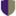Harvey Mudd College

Chip Firing Games And Riemann-Roch Properties For Directed Graphs, Joshua Z. Gaslowitz

HMC Senior Theses

The following presents a brief introduction to tropical geometry, especially tropical curves, and explains a connection to graph theory. We also give a brief summary of the Riemann-Roch property for graphs, established by Baker and Norine (2007), as well as the tools used in their proof. Various generalizations are described, including a more thorough description of the extension to strongly connected directed graphs by Asadi and Backman (2011). Building from their constructions, an algorithm to determine if a directed graph has Row Riemann-Roch Property is given and thoroughly explained.

On Toric Symmetry Of P1 X P2, 2013Harvey Mudd College

On Toric Symmetry Of P1 X P2, Olivia D. Beckwith

HMC Senior Theses

Toric varieties are a class of geometric objects with a combinatorial structure encoded in polytopes. P1 x P2 is a well known variety and its polytope is the triangular prism. Studying the symmetries of the triangular prism and its truncations can lead to symmetries of the variety. Many of these symmetries permute the elements of the cohomology ring nontrivially and induce nontrivial relations. We discuss some toric symmetries of P1 x P2, and describe the geometry of the polytope of the corresponding blowups, and analyze the induced action on the cohomology ring. We exhaustively compute the toric symmetries of P1 ...

Symbolic Powers Of Ideals In K[PN], 2013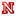University of Nebraska-Lincoln

Symbolic Powers Of Ideals In K[PN], Michael Janssen

Dissertations, Theses, and Student Research Papers in Mathematics

Let Ik[PN] be a homogeneous ideal and k an algebraically closed field. Of particular interest over the last several years are ideal containments of symbolic powers of I in ordinary powers of I of the form I(m)Ir, and which ratios m/r guarantee such containment. A result of Ein-Lazarsfeld-Smith and Hochster-Huneke states that, if Ik[PN], where k is an algebraically closed field, then the symbolic power I(Ne) is contained in the ordinary power Ie, and thus, whenever m/rN we have the containment I(m)Ir. Therefore ...

Propeller, 2013Claremont Colleges

Propeller, Joel Kahn

The STEAM Journal

This image is based on several different algorithms interconnected within a single program in the language BASIC-256. The fundamental structure involves a tightly wound spiral working outwards from the center of the image. As the spiral is drawn, different values of red, green and blue are modified through separate but related processes, producing the changing appearance. Algebra, trigonometry, geometry, and analytic geometry are all utilized in overlapping ways within the program. As with many works of algorithmic art, small changes in the program can produce dramatic alterations of the visual output, which makes lots of variations possible.

Galois Representations From Non-Torsion Points On Elliptic Curves, 2013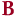Bard College

Galois Representations From Non-Torsion Points On Elliptic Curves, Matthew Phillip Hughes

Senior Projects Spring 2013

Working from well-known results regarding l-adic Galois representations attached to elliptic curves arising from successive preimages of the identity, we consider a natural deformation. Given a non-zero point P on a curve, we investigate the Galois action on the splitting fields of preimages of P under multiplication-by-l maps. We give a group-theoretic structure theorem for the corresponding Galois group, and state a conjecture regarding composita of two such splitting fields.

Fermat's Last Theorem, 2013University of Redlands

Fermat's Last Theorem, Rozalyn N. Miner

Throughout history, math has been transformed, theorems have been proven, and great people have become known through their discoveries. One of these great people was Pierre Fermat, who studied law at the University of Orleans. Through his work as a government official and the office he held there, he was able to change his name to Pierre de Fermat. There is controversy as to when Fermat was actually born. Most historians say that he was born in 1601, but through research Klaus Barner, a professor at University of Kassel, Germany, found that Fermat was most likely born in 1607. This ...

2012The College at Brockport

Geometry Curriculum For High School Students During A Summer School Program, Kyle E. Kucsmas

Education and Human Development Master's Theses

This geometry curriculum project was designed to be used during a high school summer school credit recovery program. The National Council of Teachers of Mathematics (NCTM), New York State (NYS) Learning Standards for Mathematics, Science, and Technology (MST), and the most recent addition of the Common Core Learning Standards (CCSL) harbor the foundations for each lesson. The curriculum presented will provide teachers with a condensed standards based instrument that can be utilized during a twenty-two day summer school geometry program which contains mathematical content, appropriate rigor, and opportunities for student growth. The curriculum is engaging, obtainable, cyclical, and diverse enough ...

On Hilbert Modular Threefolds Of Discriminant 49, 2012University of Massachusetts - Amherst

On Hilbert Modular Threefolds Of Discriminant 49, Lev Borisov, Paul Gunnells

Paul Gunnells

Let K be the totally real cubic field of discriminant 49 , let \fancyscriptO be its ring of integers, and let p⊂\fancyscriptO be the prime over 7 . Let Γ(p)⊂Γ=SL2(\fancyscriptO) be the principal congruence subgroup of level p . This paper investigates the geometry of the Hilbert modular threefold attached to Γ(p) and some related varieties. In particular, we discover an octic in P3 with 84 isolated singular points of type A2 .

The Secant Conjecture In The Real Schubert Calculus, 2012Sam Houston State University

The Secant Conjecture In The Real Schubert Calculus, Luis D. García-Puente, Nickolas Hein, Christopher Hillar, Abraham Martín Del Campo, James Ruffo, Frank Sottile, Zach Teitler

Zach Teitler

We formulate the Secant Conjecture, which is a generalization of the Shapiro Conjecture for Grassmannians. It asserts that an intersection of Schubert varieties in a Grassmannian is transverse with all points real if the flags defining the Schubert varieties are secant along disjoint intervals of a rational normal curve. We present theoretical evidence for this conjecture as well as computational evidence obtained in over one terahertz-year of computing, and we discuss some of the phenomena we observed in our data.

The Secant Conjecture In The Real Schubert Calculus, 2012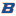Sam Houston State University

The Secant Conjecture In The Real Schubert Calculus, Luis D. García-Puente, Nickolas Hein, Christopher Hillar, Abraham Martín Del Campo, James Ruffo, Frank Sottile, Zach Teitler

Mathematics Faculty Publications and Presentations

We formulate the Secant Conjecture, which is a generalization of the Shapiro Conjecture for Grassmannians. It asserts that an intersection of Schubert varieties in a Grassmannian is transverse with all points real if the flags defining the Schubert varieties are secant along disjoint intervals of a rational normal curve. We present theoretical evidence for this conjecture as well as computational evidence obtained in over one terahertz-year of computing, and we discuss some of the phenomena we observed in our data.

Weierstrass Points On Families Of Graphs, 2012University of Connecticut

Weierstrass Points On Families Of Graphs, William D. Lindsay Jr.

Master's Theses

No abstract provided.

Communal Partitions Of Integers, 2012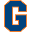Gettysburg College

Communal Partitions Of Integers, Darren B. Glass

Math Faculty Publications

There is a well-known formula due to Andrews that counts the number of incongruent triangles with integer sides and a fixed perimeter. In this note, we consider the analagous question counting the number of k-tuples of nonnegative integers none of which is more than 1/(k−1) of the sum of all the integers. We give an explicit function for the generating function which counts these k-tuples in the case where they are ordered, unordered, or partially ordered. Finally, we discuss the application to algebraic geometry which motivated this question.

2012University of Nevada, Las Vegas

The Zeta Function Of Generalized Markoff Equations Over Finite Fields, Juan Mariscal

UNLV Theses, Dissertations, Professional Papers, and Capstones

The purpose of this paper is to derive the Hasse-Weil zeta function of a special class of Algebraic varieties based on a generalization of the Markoff equation. We count the number of solutions to generalized Markoff equations over finite fields first by using the group structure of the set of automorphisms that generate solutions and in other cases by applying a slicing method from the two-dimensional cases. This enables us to derive a generating function for the number of solutions over the degree k extensions of a fixed finite field giving us the local zeta function. We then create an ...

An Investigation Into Three Dimensional Probabilistic Polyforms, 2012Olivet Nazarene University

An Investigation Into Three Dimensional Probabilistic Polyforms, Danielle Marie Vander Schaaf

Honors Program Projects

Polyforms are created by taking squares, equilateral triangles, and regular hexagons and placing them side by side to generate larger shapes. This project addressed three-dimensional polyforms and focused on cubes. I investigated the probabilities of certain shape outcomes to discover what these probabilities could tell us about the polyforms’ characteristics and vice versa. From my findings, I was able to derive a formula for the probability of two different polyform patterns which add to a third formula found prior to my research. In addition, I found the probability that 8 cubes randomly attached together one by one would form a ...

Elliptic Curves Of High Rank, 2012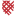Macalester College

Elliptic Curves Of High Rank, Cecylia Bocovich

Mathematics, Statistics, and Computer Science Honors Projects

The study of elliptic curves grows out of the study of elliptic functions which dates back to work done by mathematicians such as Weierstrass, Abel, and Jacobi. Elliptic curves continue to play a prominent role in mathematics today. An elliptic curve E is defined by the equation, y2 = x3 + ax + b, where a and b are coefficients that satisfy the property 4a3 + 27b2 = 0. The rational solutions of this curve form a group. This group, denoted E(Q), is known as the Mordell-Weil group and was proved by Mordell to be isomorphic to Zr ⊕ E ...

A Multiplicative "Conic", 2012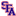Stephen F Austin State University

A Multiplicative "Conic", Tiffany Lundy

Early in their mathematical career, students learn about how ellipses and hyperbolas are formed, their properties, and their applications. In particular, an ellipse is the set of all points in the plane whose combined distance from two fixed locations (foci) is constant. A hyperbola is formed in much the same way, except instead of combining (adding) the distance from the foci, the difference is used. This research begins by examining a new locus of points. The locus of points if formed by taking all points whose distance from two fixed locations when multiplied in constant. The difference discernible types of ...

Non-Genera Of Curves With Automorphisms In Characteristic P, 2012Gettysburg College

Non-Genera Of Curves With Automorphisms In Characteristic P, Darren B. Glass

Math Faculty Publications

We consider which integers g and r can occur respectively as the genus and p-rank of a curve defined over a field of odd characteristics p which admits an automorphism of degree p.

Road Trips In Geodesic Metric Spaces And Groups With Quadratic Isoperimetric Inequalities, 2011Eastern Kentucky University

Road Trips In Geodesic Metric Spaces And Groups With Quadratic Isoperimetric Inequalities, Rachel Bishop-Ross, Jon Corson

Rachel E. Bishop-Ross

We introduce a property of geodesic metric spaces, called the road trip property, that generalizes hyperbolic and convex metric spaces. This property is shown to be invariant under quasi-isometry. Thus, it leads to a geometric property of finitely generated groups, also called the road trip property. The main result is that groups with the road trip property are finitely presented and satisfy a quadratic isoperimetric inequality. Examples of groups with the road trip property include hyperbolic, semihyperbolic, automatic and CAT(0) groups. DOI: 10.1142/S0218196712500506

Mixed Discriminants, 2011University of Massachusetts Amherst

Mixed Discriminants, Eduardo Cattani, Maria Angelica Cueto, Alicia Dickenstein, Sandra Di Rocco, Bernd Strumfels

Eduardo Cattani

No abstract provided.

Infinitary Equivalence Of Zp- Modules With Nice Decomposition Bases, 2011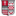Universitat Duisburg-Essen, Germany

Infinitary Equivalence Of Zp- Modules With Nice Decomposition Bases, Rüdiger Göbel, Katrin Leistner, Peter Loth, Lutz Strüngmann

Mathematics Faculty Publications

Warfield modules are direct summands of simply presented Zp - modules, or, alternatively, are Zp - modules possessing a nice decomposition basis with simply presented cokernel. They have been classified up to isomorphism by theor Ilm-Kaplansky and Warfield invariants. Taking a model theoretic point of view and using infinitary languages we give here a complete theoretic characterization of a large class of Zp - modules having a nice decomposition basis. As a corollary, we obtain the classical classification of countable Warfield modules. This generalizes results by Barwise and Eklof.